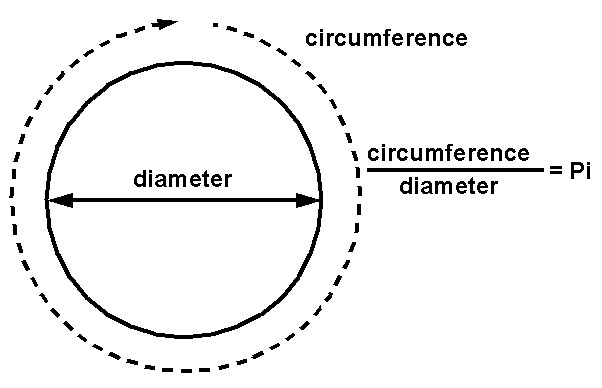## Pi Instant: March 14, 2015, at 9:26:53.58979... a.m.The mathematical constant pi (or π) represents the ratio of a circle's circumference to its diameter. It is an extremely important value for geometry, trigonometry, calculus, Fourier analysis, quantum mechanics, and so much more. It has no exact rational or finite decimal form, but its numerical value is approximately 3.1415926535897932385...

In honour of pi, Pi Day is celebrated each March 14, since "March 14" can be written as "3/14" which comprises the first three digits of pi. Pi Day in the year 2015 will be special, since "March 14, 2015" could be written as "3/14/15", thus comprising the first five digits of pi, not just three. Extending this, some have observed that on March 14, 2015, at the time 9:26:53 a.m. (or p.m.), the date and time could be written as "3/14/15; 9:26:53", thus comprising the first ten digits of pi -- a "Pi Second".

I propose to take this idea a step further. The time 9:26:53 refers to 26 minutes and 53 seconds after 9 o'clock. By extension, the time 9:26:53.58979... refers to 26 minutes and 53.58979... seconds after 9 o'clock. If the remaining (infinite) decimal expansion of 53.58979... is chosen to follow pi's digits exactly, then we obtain a single precise instant, "3/14/15; 9:26:53.58979...", during which all of the infinite digits of pi are all represented by a single precise date and time.

I thus propose that March 14, 2015, at 9:26:53.58979... (i.e., at 26 minutes and 53.58979... seconds after 9 o'clock a.m., where the remaining digits of 53.58979... all follow the digits of pi) be designated and celebrated as Pi Instant, the single instant when all of pi's infinite digits are all laid out before us. Start planning your parties now!

-- Jeffrey S. Rosenthal, October 2014
(A slightly condensed version of this note was published in the February 2015 issue of Math Horizons; see also a related radio interview and my note about piems.)